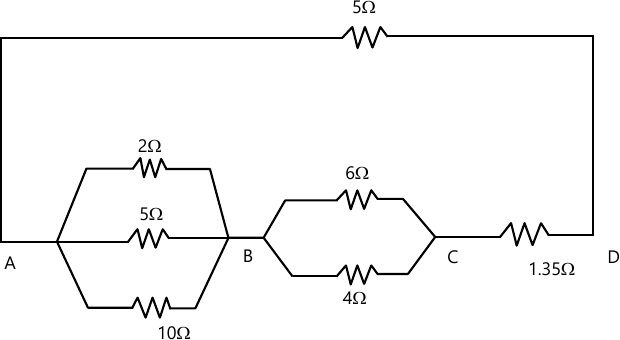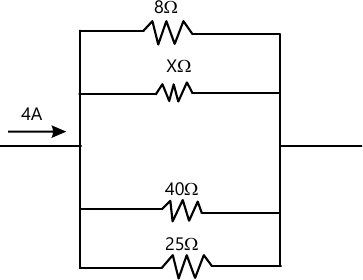MORE IN Basic Electrical Engg.
VTU First Year Engineering (P Cycle) (Semester 2)
Basic Electrical Engg.
June 2013
Total marks: --
Total time: --
INSTRUCTIONS
(1) Assume appropriate data and state your reasons
(2) Marks are given to the right of every question
(3) Draw neat diagrams wherever necessary

Choose the correct answers for the following
1(a)(i) The condition for the validity under Ohm's law is that the
a) Temperature should remain constant b) Current should be proportional to voltage c) Resistance must be wire wound type d) all of the above
1 M
1(a)(ii) A linear resister is one which obeys
a) Ampere's law b) Lenz's law c) Ohms law cd Kirchhoff s law
1 M
1(a)(iii) The resistance of a conductor having length l, area of cross section a and resistivity ρ is given as
a) R=ρa/l b) R=ρl/a c) R=ρla d) R=l/aρ
1 M
1(a)(iv) Resistance of a wire always increases if
a) Temperature is reduced b) Temperature is increased c) Number of free electrons available become less d) Number of free electrons available become more.
1 M
1(b) Find the resistance of the circuit shown5 M
1(c) State and explain Kirchhoff's laws
5 M
1(d) In the parallel arrangement of resistors shown, the current flowing in the 8Ω resistor is 2.5A. Find :
(i) Current in other resistors
(ii) Resistor X
(iii) The equivalent resistance6 M

Choose the correct answers for the following
2(a)(i) The law that finds application in electrolysis
a) Faraday's law b) Coulomb's law c) Ohm's law d) Lenz's law
1 M
2(a)(ii) According to Faraday's law of electromagnetic induction an emf is induced in a conductor whenever it
a) Lies in a magnetic field b) lies perpendicular to the magnetic field c) Cuts the magnetic flux d) Moves parallel to the direction of magnetic field.
1 M
2(a)(iii) "In all cases of electromagnetic induction, an induced voltage will cause a current to flow in a closed circuit in such a direction that the magnetic field which is caused by that current will oppose the change that produces the current" is the original statement of
a) Lenz's law b) Faraday's law of magnetic induction c) Fleming's law of induction d) Ampere's law
1 M
2(a)(iv) Which law is synonymous to the occurrence of diamagnetism?
a) Ampere's law b) Maxwell's law c) Coulomb's law d) Lenz's law.
1 M
2(b) State and explain faradays laws of electromagnetic induction.
8 M
2(c) Derive the expression for energy stored in an inductor.
8 M

Choose the correct answers for the following
3(a)(i) The form factor is the ratio of
a) Average value to rms value b) Rms value to average value c) Peak value to average value d) Peak value to rms value
1 M
3(a)(ii) In an R - L series circuit the pf is
a) Leading b) Lagging c) Zero d) Unity
1 M
3(a)(iii) The power factor of an AC circuit is equal to
a) cosine of the angle b) Sine of the phase angle c) Unity for a resistive circuit d) Unity for a reactive circuit
1 M
3(a)(iv) In a pure capacitive circuit, the current will
a) Lag behind the voltage by 90° b) Lead the voltage by 90° c) Remain in phase with voltage d) None of these
1 M
3(b) Derive an expression for the impedance of an AC circuit consisting of a resistance an inductance and a capacitance connected in series.
10 M
3(c) 125 volts at 60Hz is applied across a capacitance connected in series with a non-inductive resistor. The combination carries a current of 2.2A and causes a power loss of 96.8 W in the resistor. Power loss in the capacitor is negligible. Calculate the resistance and capacitance.
6 M

Choose the correct answers for the following
4(a)(i) In a 3 phase balanced star - connected load, neutral current is equal to
a) Zero b) IP c) IL d) Unpredictable
1 M
4(a)(ii) The relationship between the line and phase voltage of a delta connected circuit is given by
1 M
4(a)(iii) The power in a 3 phase system is given by EQUATION where ? is the phase angle between
a) Line voltage and line current b) Phase voltage and phase current c) Line voltage and phase current d) Phase voltage and line current
1 M
4(a)(iv) Three equal impedances are first connected in delta across a 3 - phase balanced supply. If the same impedances are connected in star across the same supply
a) Phase currents will be one - third b) Line currents will be one - third c) Power consumed will be one - third d) None of these
1 M
4(b) Derive the relation between a line current and a phase current and a line voltage and phase voltage related to a star connected load.
7 M
4(c) Mention the different types of wiring used in domestic dwellings.
3 M
4(d) Explain the construction and working of induction type single phase energy meter.
6 M

PART B:
Choose the correct answers for the following
5(a)(i) The emf generated in a dc generator depends upon
a) Brush contact drop b) Commutation c) Number of parallel paths d) Terminal voltage
1 M
5(a)(ii) The dc generator having residual magnetism gives zero induced emf. The speed will be
a) Zero b) Very small c) Rated one d) Any
1 M
5(a)(iii) The field coils of a dc machine are made of
a) Carbon b) Copper c) Mica d) Steel
1 M
5(a)(iv) The rotating part of a dc machine is called the
a) Rotor b) Field c) Armature d) Stator
1 M
5(b) The emf generated in the armature of a shunt generator is 625 V, when delivering its full load current of 400 A to the external circuit. The field current is 6 A and the armature resistance is 0.06?. What is the terminal voltage?
8 M
5(c) A 220 volts series motor is taking a current of 40 A. Resistance of armature 0.5Ω, resistance of series field is 0.25Ω. Calculate :
(i) Voltage at the brushes
(ii) Back emf
(iii) Power wasted in armature
(iv) Power wasted in series
8 M

Choose the correct answers for the following
6(a)(i) Transformer is used
a) To step up the voltage b) To step down the voltage c) On dc d) To step up or step down the voltage
1 M
6(a)(ii) A transformer does not transform
a) Power b) Voltage c) Current d) Impedance
1 M
6(a)(iii) In a transformer, electrical power is transferred from primary to secondary
a) Through air b) By magnetic flux c) Through insulating medium d) None of these
1 M
6(a)(iv) The two windings of a transformer are
1 M
6(b) Explain principle of operation of a single phase transformer and derive the EMF equation.
8 M
6(c) A single phase, 20 KVA transformer has 1000 primary turns and 2500 secondary turns. The net cross sectional area of the core is 100cm2 . When the primary winding is connected to 500V, 50Hz supply, Calculate :
(i) The maximum value of the flux density in the core
(ii) The voltage induced in the secondary winding and
(iii) The primary and secondary full load currents.
8 M

Choose the correct answers for the following
7(a)(i) In a synchronous machine, the stator frame is made of
a) Stain steel b) CRC0GS c) Cast iron or welded steel plates d) Laminated silicon steel
1 M
7(a)(ii) The stator core of a synchronous machine is laminated so as to reduce,
a) Eddy current loss b) Hysteresis c) Both eddy current and hysteresis loss d) The size and weight of the machine
1 M
7(a)(iii) The stator slot insulations in synchronous made of.
a) Mica cloth b) Fibre glass c) Polyester sheets d) Any of these
1 M
7(a)(iv) The machine that supplies dc to the rotor is called the,
a) Rectifier b) Exciter c) Convertor d) Invertor
1 M
7(b) Derive EMF equation of an alternator
8 M
7(c) Explain construction and working principle of synchronous generator
8 M

Choose the correct answers for the following
8(a)(i) If a single phase induction motor runs at a speed lower than the rated one, the most likely defect is,
a) Improper size fuses b) Worn-out bearings or low voltage or over load c) Open-circuit in the winding d) Short-circuit in the winding
1 M
8(a)(ii) If the starting winding of a single phase induction motor is left in the circuit.
a) The motor will run faster b) The motor will run slower c) There will be undue sparking d) The auxiliary winding will get over-heated due to continuous flow of current and may get damaged.
1 M
8(a)(iii) Which of the following types of motors are not single phase ac motors?
a) Induction type motors b) Commutator type motors c) Synchronous type motors d) Schrage motors
1 M
8(a)(iv) Which of the following types of motors are not the induction motors?
a) Repulsion motors b) Split phase motors c) Shaded pole motors d) Repulsion start induction motors
1 M
8(b) Explain construction and working principle of star-delta starter.
8 M
8(c) What is meant by the slip of the induction motor? Under what circumstances the slip is
(i) Unity and
(ii) Zero
8 M

More question papers from Basic Electrical Engg.